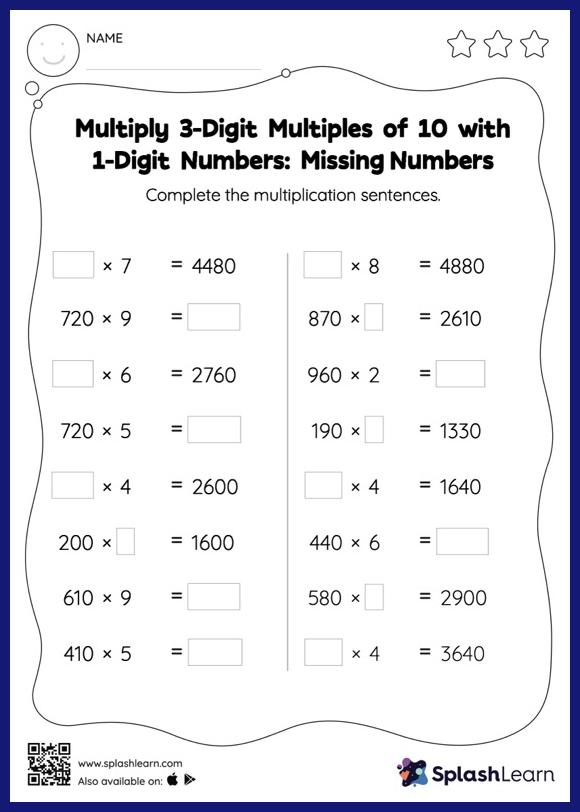# Multiply 3-Digit Multiples of 10 with 1-Digit Numbers: Missing Numbers Worksheet

Home > Multiply 3-Digit Multiples of 10 with 1-Digit Numbers: Missing NumbersHelp your little one develop a knack for math with this multiply 3-digit multiples of 10 with 1-digit numbers worksheet. Students love it when they get to multiply numbers by 10, 100, or their multiples. They use the pattern of zeros to find the product. In multiply 3-digit multiples of 10 with 1-digit numbers worksheet, they use the same concept to find the missing number in multiplication sentences.